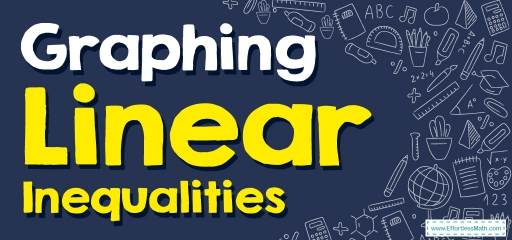# How to Graph Linear Inequalities? (+FREE Worksheet!)

Learn how to graph linear inequalities using a testing point in a few simple steps.## Step by step guide to graphing linear inequalities

• First, graph the “equals” line.
• Choose a testing point. (it can be any point on both sides of the line.)
• Put the value of $$(x, y)$$ of that point in the inequality. If that works, that part of the line is the solution. If the values don’t work, then the other part of the line is the solution.

The Absolute Perfect Book to Ace the College Algebra Course

### Graphing Linear Inequalities – Example 1:

Sketch the graph of $$y<2x-4$$.

Solution:

First, graph the line:
$$y=2x-4$$. The slope is $$2$$ and $$y$$-intercept is $$-4$$.
Then, choose a testing point. The easiest point to test is the origin: $$(0,0)$$
$$(0,0)→y<2x-4→0<2(0)-4→0<-4$$
$$0$$ is not less than $$-4$$. So, the other part of the line (on the right side) is the solution.

### Graphing Linear Inequalities – Example 2:

Sketch the graph of $$y<2x-3$$.

Solution:

First, graph the line:
$$y=2x-3$$. The slope is $$2$$ and $$y$$-intercept is $$-3$$.
Then, choose a testing point. The easiest point to test is the origin: $$(0,0)$$
$$(0,0)→y<2x-3→0<2(0)-3→0<-3$$
$$0$$ is not less than $$-3$$. So, the other part of the line (on the right side) is the solution.

## Exercises for Graphing Linear Inequalities

### Sketch the graph of each linear inequality.

• $$\color{blue}{y < – 4x + 2}$$
• $$\color{blue}{2x + y < – 4}$$

• $$\color{blue}{y < – 4x + 2}$$
• $$\color{blue}{2x + y < – 4}$$

The Absolute Best Books You Need to Ace Algebra

### What people say about "How to Graph Linear Inequalities? (+FREE Worksheet!) - Effortless Math: We Help Students Learn to LOVE Mathematics"?

No one replied yet.

X
45% OFF

Limited time only!

Save Over 45%

SAVE $40 It was$89.99 now it is \$49.99